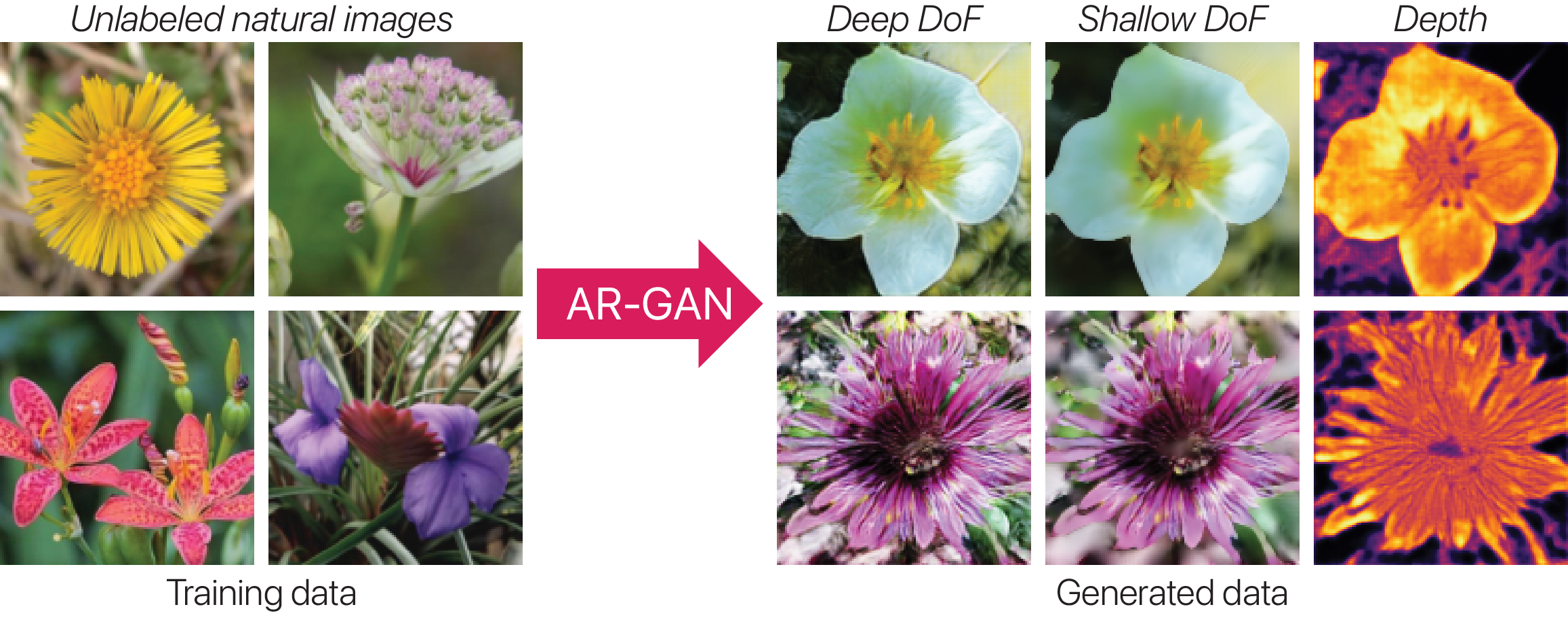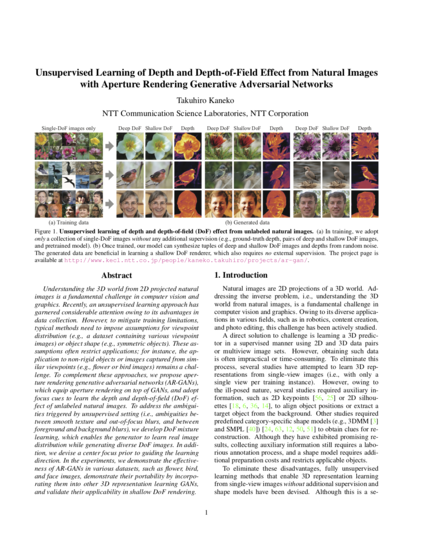### with Aperture Rendering Generative Adversarial Networks

CVPR 2021 (Oral)
[Paper] [Slides] [Poster]
[News Release (Japanese)]

We propose aperture rendering GANs (AR-GANs), which equip aperture rendering on top of GANs and adopt focus cues to learn the depth and depth-of-field (DoF) effect of unlabeled natural images.Figure 1. Unsupervised learning of depth and DoF effect from unlabeled natural images. (a) In training, we adopt only a collection of single-DoF images without any additional supervision (e.g., ground-truth depth, pairs of deep and shallow DoF images, and pretrained model). (b) Once trained, AR-GANs can synthesize tuples of deep and shallow DoF images and depths from random noise.

### Abstract

Understanding the 3D world from 2D projected natural images is a fundamental challenge in computer vision and graphics. Recently, an unsupervised learning approach has garnered considerable attention owing to its advantages in data collection. However, to mitigate training limitations, typical methods need to impose assumptions for viewpoint distribution (e.g., a dataset containing various viewpoint images) or object shape (e.g., symmetric objects). These assumptions often restrict applications; for instance, the application to non-rigid objects or images captured from similar viewpoints (e.g., flower or bird images) remains a challenge. To complement these approaches, we propose aperture rendering generative adversarial networks (AR-GANs), which equip aperture rendering on top of GANs, and adopt focus cues to learn the depth and DoF effect of unlabeled natural images. To address the ambiguities triggered by unsupervised setting (i.e., ambiguities between smooth texture and out-of-focus blurs, and between foreground and background blurs), we develop DoF mixture learning, which enables the generator to learn real image distribution while generating diverse DoF images. In addition, we devise a center focus prior to guiding the learning direction. In the experiments, we demonstrate the effectiveness of AR-GANs in various datasets, such as flower, bird, and face images, demonstrate their portability by incorporating them into other 3D representation learning GANs, and validate their applicability in shallow DoF rendering.

### Aperture rendering GANs: AR-GANs

In the following, we first explain the overall pipeline of AR-GAN generator, and then describe two key ideas, i.e., DoF mixture learning and the center focus prior.

#### 1. Overall pipeline

The overall pipeline of the AR-GAN generator is illustrated in Figure 2. The AR-GAN generator (1) generates a deep DoF image $$I_d^g$$ and depth $$D^g$$ from a random noise $$z$$, (2) simulates the light field $$L^g$$ by warping $$I_d^g$$ based on $$D^g$$, and (3) renders a shallow DoF image $$I_s^g$$ by integrating $$L^g$$ using the aperture $$A$$.

#### 2. DoF mixture learning

Figure 3 illustrates the comparison between standard and DoF mixture learning. (a) In standard GAN training, the generator attempts to cover the real image distribution using images without constraints. (b) In contrast, in the DoF mixture learning, the generator attempts to represent the real image distribution using diverse DoF images whose extent is adjusted by a scale factor $$s$$. Intuitively, the aperture renderer $$R$$, which has an optical constraint on the light field, functions as a shallow DoF image prior. This prior encourages the generated deep $$I_d^g$$ and shallow $$I_s^g$$ DoF images to be approximate to real deep $$I_d^r$$ and shallow $$I_s^r$$ DoF images, respectively. This prior also facilitates the learning of $$D^g$$, which is a source of the $$I_d^g$$ and $$I_s^g$$ connection.

#### 3. Center focus prior

Another challenge unique to unsupervised depth and DoF effect learning is to the difficulty in distinguishing foreground and background blurs without any constraint or prior knowledge. Although not all images satisfy this, focused images tend to be captured when the main targets are positioned at the center, as shown in Figure 4 (a). Based on this observation, we impose a center focus prior, which acilitates the center area focus while promoting the surrounding area to be behind the focal plane. We visualize this prior in Figure 4 (b).

### Example results

#### Examples of generated data

We evaluated AR-GANs on three natural image datasets that cover various objects: flower (Oxford Flowers), bird (CUB-200-2011), and face (FFHQ) datasets. We present the results for these datasets in Figures 5, 6, and 7, respectively.

#### Portability analysis

AR-GAN has portability and can be easily incorporated into viewpoint-aware GANs (e.g., RGBD-GAN [Noguchi et al., 2020]). Examples of data generated using AR-RGBD-GAN (i.e., AR-GAN + RGBD-GAN) are shown in Figure 8. In this figure, the viewpoint change in the horizontal direction is obtained by the RGBD-GAN function, whereas the DoF change and depth in the vertical direction are obtained by the AR-GAN function.

After training, AR-GAN can synthesizes tuples of $$( I_d^g, I_s^g, D^g )$$ from random noise. By utilizing this, we learn a depth estimator $$I_d \rightarrow D$$ using pairs of $$(I_d^g, D^g)$$. By employing the learned depth estimator, we estimate $$D$$ from $$I_d$$ and then render $$I_s$$ from $$(I_d, D)$$ using the aperture render $$R$$ in AR-GAN. We call this approach AR-GAN-DR. Examples of rendered images are presented in Figure 9. We found that CycleGAN often yields unnecessary changes (e.g., color change), whereas AR-GAN-DR does not. We infer that the aperture rendering mechanism in AR-GAN contributes to this phenomenon. In addition, AR-GAN-DR can estimate the depth simultaneously.Takuhiro Kaneko. Unsupervised Learning of Depth and Depth-of-Field Effect from Natural Images with Aperture Rendering Generative Adversarial Networks. In CVPR, 2021. (arXiv:2106.13041, June 2021) [Paper] [BibTex]